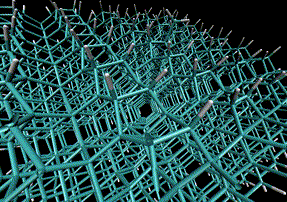# dynamics at diamond surfacesWe’ve just published a paper (open-access link here) looking at non-equilibrium reaction dynamics at the surface of diamond. As shown in the video, our simulations enabled us to look at the dynamics of a slab of hydrogen capped diamond.

We constructed the diamond slab so as to contain a single “dangling” surface methyl group. Diamond is a notoriously good heat conductor: from a microscopic perspective, this means that it dissipates vibrational energy extremely efficiently. So we were surprised to observe that placing some initial heat into the dangling CH3 group (by “plucking” its bonds) led to a statistically significant number of dissociation events. Not an enormous number of events, but enough to matter.

The conventional knowledge is that such events essentially have a probability of zero – owing to the fact that diamond is so efficient at heat dissipation. So we set out to understand why we observed any dissipation. To do so, we formulated an energy-grained master equation model, an area where I’ve been active for while. To some extent, this approach followed on from work we carried out back in 2009, which was aimed at understanding the competition between reaction and relaxation dynamics in organic solvents. Our 2009 study was the first to apply the energy grained master equation to reactions in condensed phases; and to the best of my knowledge, the diamond work represents the first attempt to apply the energy grained master equation to reactions at surfaces.

The big problem with a master equation representation of diamond arises from the fact that it’s not straightforward to separate the “system” degrees of freedom from the “bath” degrees of freedom. For reactions in liquids, the separation is somewhat more straightforward: it is effectively the solute/solvent distinction. For diamond, we simply tried a sensible definition: let the “system” be defined as those atoms and corresponding vibrations which were less than 3 covalent bonds away from the constituent methyl group; and let the “bath” be defined as everything else. This allowed us to calculate energy resolved rate coefficients [k(E)s] for CH3 dissociation from the “system” component of the diamond surface. The next issue a master equation approach faces is this: how do we represent the energy transfer rate from the diamond “system” to the “bath”? Our approach was to run a single long trajectory of the diamond slab (as shown in the video), and then use linear response theory to analyze the characteristic timescale for energy fluctuations within the diamond “system” to dissipate into the “bath”. Then we parameterised an energy transfer function to fit the energy decay curve. With these two ingredients – k(E)s, and an energy transfer function – we could run master equation simulations of the surface reaction dynamics. The results showed the energy dissipation from the system to the bath is definitely fast (with a timescale of ~100 fs) but that there is indeed a non-trivial probability that “prompt” CH3 dissociation events occur – i.e., prior to dissipation of all the energy.

Similar to the 2009 results, we actually found that the master equation did a pretty decent job compared to full MD simulations of CH3 dissociation! But for a computational cost which is 1/100,000 the cost of running the full set of dissociative MD simulations – big savings! As the first of its kind, this work is preliminary in many important respects, but it definitely offers a viable option for relatively cheap modelling of non-equilibrium reaction dynamics at surfaces. Note that all of the input files required to run the model are being made available in MESMER, our cross-platform, open-source master equation solver.